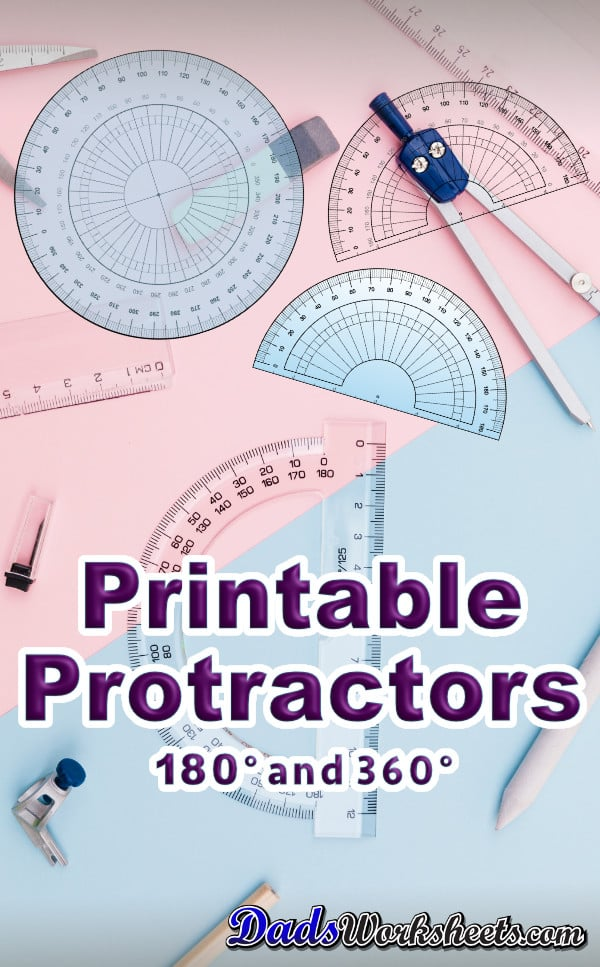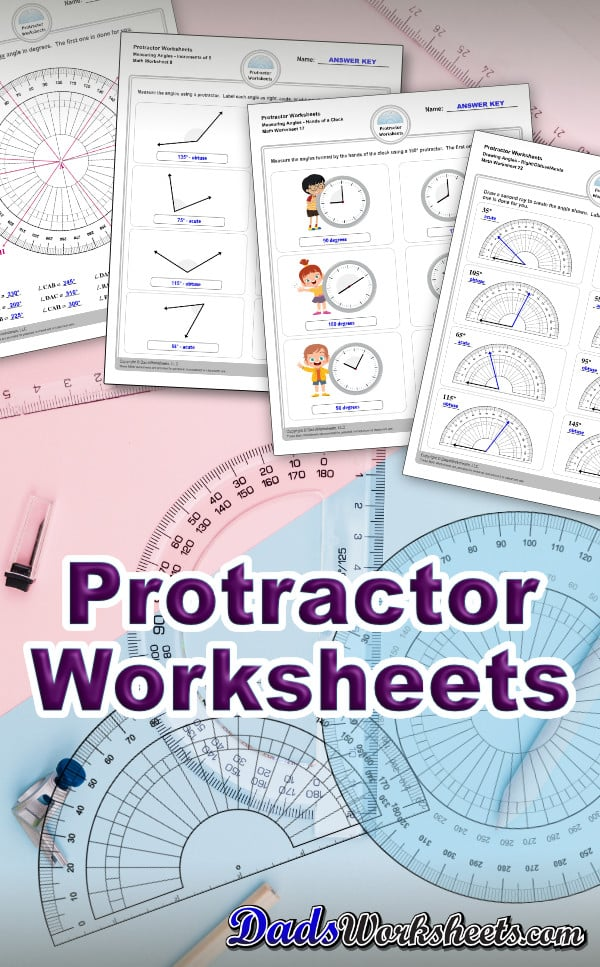# Angles with a Protractor

The protractor worksheets and blank printable projectors on this page require students to measure angles and identify whether they are right, acute or obtuse. The worksheets start out with the base leg of the angle always laying horizontal, which is the easiest way to visualize whether the angle is right, acute, or obtuse. In later worksheets in the set, students measure angles with a protractor and supply the measurements as answers to the problems.

Some of the worksheets have the base angle rotated slightly which requires more spatial thinking, and the other set of worksheets require students to measure reflex angles, read the hands of a clock, draw angles and more! All of these PDF worksheets include answer keys.

Each worksheet in this section provide great practice for measuring angles with a protractor. If you don’t have a protractor on hand, you may download a printable protractor from this page as well.We have created different kinds of protractors — the traditional semi-circle 180° protractor or the full circle 360° protractor, both available in black and white or colored.

## How To Use a Protractor

Before we proceed with the steps on how to use a protractor, let’s discuss first what this tool is.A protractor is a tool used for measuring angles in degrees.The protractor is usually semi-circle size divided into 180 parts or a full circle divided into 360 parts. It is usually made of transparent glass or transparent plastic, while some are made of wood, ivory, or metal. Protractors usually have two sets of numbers going in opposite directions. The outer scale runs from 0 to 180 or 0 to 360 degrees going clockwise. The inner scale runs from 0 to 180 or 0 to 360 degrees going anticlockwise. The images below shows the most commonly used protractors in school for measuring angles in geometry class.Moving forward, here are the steps on how to use a protractor.Let’s use the traditional protractor with outer scale of 0-180 degrees on our example below.

Step 1: Find the vertex of the angle. Put the hole, circle, or dot of your protractor so that it’s lined up with the vertex.This might also look like a a little upside down letter “T” in some protractors.Step 2: Line one of the rays with the line at the bottom of the protractor where you see the number “0”.The bottom ray should be on the zero edge and the vertex is in the center point or hole of the protractor.Step 3: See where the other ray intersects the protractor's scale. Read the degrees where the other side crosses the number scale. That’s where you will find the angle measurement.You may have to extend the line of the second ray in some cases to determine the exact measurement of the angle.The angle below measures 90° or 90 degrees.### Using a 360 Degree Protractor

Let’s use a 360° protractor this time.It is circular in shape and has readings up to 360° in clockwise direction for outer scale and inner scale going anticlockwise or the opposite direction.

Step 1: Find the vertex of the angle. Put the hole, circle, or dot of your protractor so that it’s lined up with the vertex.Step 2: Place one of the rays within the line at the zero edge where you see the number “0” and the vertex at the center point or hole of the protractor.

Note:You’ll notice two sets of degrees along the edge: an inner and outer scale. Both scales go from 0 to 360, but they run in opposite directions. If the angle opens to the right side of the protractor, use the inner scale. If the angle opens to the left of the protractor, use the outer scale.

Let’s use the outer scale on our example.Step 3: See where the other ray intersects the protractor's scale. This is similar to when using a 180° protractor, and you may have to extend the line of the second ray in some cases to determine the exact measurement of the angle. The angle below measures 230° or 230 degrees.### Measuring Angles with a Protractor

An angle is the distance, measured in degrees, between two lines that share one endpoint ~ these lines are called rays. When two rays intersect, at the point of their intersection an angle is formed. When measuring the angle, one arm is fixed as the base while the other moves clockwise or counterclockwise to form an angle between the two. Therefore, all angles have two “sides” or “arms” or “ rays” and one “vertex”. The exact degree of the angle is measured by a protractor or calculated based on other angles. As an angle increases, the name of the angle changes. Let’s discuss in detail the different types of angles based on their measurements. Refer to the image below.### Right Angle

A right angle is exactly 90 degrees. Imagine the corner of a book or the corner of a square or rectangular table. The two lines are perfectly perpendicular to each other. If one line is horizontal, the right angle is created by drawing a second line 90 degrees away from it. A right angle is indicated by drawing a small square in the angle, as shown in the image above. Keep this in mind: A right angle is equivalent to 1/4 turn of a circle. A full circle is exactly 360 degrees and 1/4 of it is equivalent to 90 degrees.

### Acute Angle

Acute angles are less than 90 degrees, but more than zero degrees. This angle is smaller than the right angle, which is exactly 90 degrees. Refer to the shaded blue angles in the image above, notice the small spaces from 0 and 90 degree lines indicating that acute angles are less than 90 degrees, but more than zero degrees. A “V” shape is an example of an acute angle.

### Obtuse Angle

Obtuse angles are more than 90 degrees, but less than 180 degrees. Obtuse angle is the opposite of acute angle. The image above shows exactly how they are opposite to each other (blue and green angles). Another tip to remember, you can make an obtuse angle by drawing a right angle and then adding more degrees to the second line.

### Straight Angle

A straight angle is exactly 180 degrees. This is half a circle. A full circle is 360 degrees total. A straight angle can also be called a straight line. The straight line of a ruler is one example of straight angle.

### Reflex Angle

A reflex angle is more than 180 degrees, but less than 360 degrees or one turn, or an entire circle. It will look like a piece or slice taken out of a pie, where the remaining pie is the reflexive angle. Angles such as 190 or 230 degrees which are more than 180 but less than 360 degrees are called reflex angles.

### Complete Angle

A 360 degree angle or a full circle is called a complete angle, also sometimes called a full angle. It starts at 0 degree and goes all the way around for 360 degrees until it is at 0 degree again. Example of a complete angle is a pie or a round coin that makes a complete 360 degree angle.

There you have it, the different types of angles. Learning angles proved to be difficult for some. But in the end, with continuous practice on how to measure them, it will provide you with an invaluable opportunity to see how angles are an essential and important part of the real world.